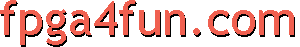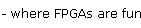## Serial interface 2 - Baud generator

Here we want to use the serial link at maximum speed, i.e. 115200 bauds (slower speeds would also be easy to generate). FPGAs usually run at MHz speeds, well above 115200Hz (RS-232 is pretty slow by today's standards). We need to find a way to generate (from the FPGA clock) a "tick" as close as possible to 115200 times a second.

Traditionally, RS-232 chips use a 1.8432MHz clock, because that makes generating the standard baud frequencies very easy... 1.8432MHz divided by 16 gives 115200Hz.

 // let's assume the FPGA clock signal runs at 1.8432MHz // we create a 4-bit counter reg [3:0] BaudDivCnt; always @(posedge clk) BaudDivCnt <= BaudDivCnt + 1; // count forever from 0 to 15 // and a tick signal that is asserted once every 16 clocks (so 115200 times a second) wire BaudTick = (BaudDivCnt==15);

That was easy. But what do you do if instead of 1.8432MHz, you have a 2MHz clock? To generate 115200Hz from a 2MHz clock, we need to divide the clock by "17.361111111..." Not exactly a round number. The solution is to divide sometimes by 17, sometimes by 18, making sure the ratio stays "17.361111111". That's actually easy to do.

Look at the following "C" code:

 while(1) // repeat forever {   acc += 115200;   if(acc>=2000000) printf("*"); else printf(" ");   acc %= 2000000; }

That prints the "*" in the exact ratio, once every "17.361111111..." loops on average.

To obtain the same thing efficiently in an FPGA, we rely on the fact that the serial interface can tolerate a few % of error in the baud frequency generator.

It is desirable that the 2000000 be a power of two. Obviously 2000000 is not. So we change the ratio... Instead of "2000000/115200", let's use "1024/59" = 17.356. That's very close to our ideal ratio, and makes an efficient FPGA implementation: we use a 10-bit accumulator incremented by 59, with a tick marked everytime the accumulator overflows.

 // let's assume the FPGA clock signal runs at 2.0000MHz // we use a 10-bit accumulator plus an extra bit for the accumulator carry-out reg [10:0] acc;   // 11 bits total! // add 59 to the accumulator at each clock always @(posedge clk)   acc <= acc[9:0] + 59; // use 10 bits from the previous accumulator result, but save the full 11 bits result wire BaudTick = acc; // so that the 11th bit is the accumulator carry-out

Using our 2MHz clock, "BaudTick" is asserted 115234 times a second, a 0.03% error from the ideal 115200.

##### Parameterized FPGA baud generator

The previous design was using a 10 bits accumulator, but as the clock frequency increases, more bits are required.

Here's a design with a 25MHz clock and a 16 bits accumulator. The design is parameterized, so easy to customize.

 parameter ClkFrequency = 25000000; // 25MHz parameter Baud = 115200; parameter BaudGeneratorAccWidth = 16; parameter BaudGeneratorInc = (Baud<

One last implementation issue: the "BaudGeneratorInc" calculation is wrong, due to the fact that Verilog uses 32 bits intermediate results, and the calculation exceeds that. Change the line as follow for a workaround.

 parameter BaudGeneratorInc = ((Baud<<(BaudGeneratorAccWidth-4))+(ClkFrequency>>5))/(ClkFrequency>>4);